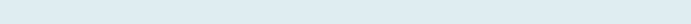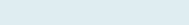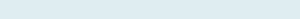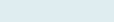# To get Hours or Minute between Two Time

In this article, you are going to learn time difference i.e. how to add or subtract between two times and to get negative time to remove ## in a cell. You can also extract hours, minutes and seconds from the given time.

Query: We have three columns Name, Start_time and End_time. Our task is to know how much time each user work including minutes and seconds. So basically find the difference of two times.

Formula to implement:

=IF(D5>C5, D5-C5, D5+C5)

Functions applicable:

The Excel IF function is a handy logical operator that returns a value based on a valid test of criteria, if it matches (TRUE) and another value if it does not.

Syntax

= IF(logical_test, value_if_true, [value_if_false])

Parameters

logical_test – The criteria to evaluate.
value_if_true [optional] – The value to return if criteria match (TRUE).
value_if_false – [optional] – The value to return if criteria does not match (FALSE).

Formula explanation:

=IF(D5>C5, D5-C5, D5+C5)

Result: 6:00:40

If the end time greater than start time then it will return hours, minutes and seconds after subtracting other than it will add two times together in a cell.

The same way you can get the same time by applying another formula with TEXT adding custom format.

Formula to implement:

=TEXT(D5-C5,”h:mm:ss”)

Result: 6:00:40

So, by this, you can get time difference with the use of any of the both to know the exact time taken by the user after knowing start and end time.

## Time difference without colon (“:”)

You may have time without colon (“:”) to properly format we can use Excel function to substitute part of a text string. And then find the difference between both times. Excel returns numbers series i.e. time value, not in minute. So to find value in minute we can simply add custom format as [m], the easy way. We can add other formats as well to find different values between time with Hour, Minute or Seconds.

Now Excel return minutes rather than numbers. We can also use  TEXT function for converting a value to a specific format.

## Negative Time Error

When you get result of time in the negative form it returns (##) in the cell to remove such time value i.e. to show negative time in Excel.

Manage Errors with formula below.

=TEXT(MAX(C3:D3)-MIN(C3:D3),”-h:mm:ss”)

Function applicable

Text: The Excel TEXT function allows converting a value to a specific format based on the given format codes. The function is useful to display text after inserting a given number formatting.

Max: The Excel MAX function returns the numerical with the highest value as per given reference of cell range.

Min: The Excel MIN function returns the smallest numerical with the lowest value as per given reference of cell range.

Formula explanation step by step:

Let’s break the formula into multiple small steps to understand its application.

First formula:Syntax: =MAX (number1, [number2], …)

=MIN (number1, [number2], …)

Step1:Result: 0.125

It will first show time then subtract large time with small time from the given time.

Second formula:Syntax: =TEXT (value, format_text)

Step 2:Result: -3:00:00

It will return negative time as bigger time with smaller after subtracting in “h:mm:ss” format.

Remember: You can also convert the format of time like 24 to 12 hours and so on.